# Algorithms CS 202 Epp section Aaron Bloomfield 1

• Slides: 25
Download presentation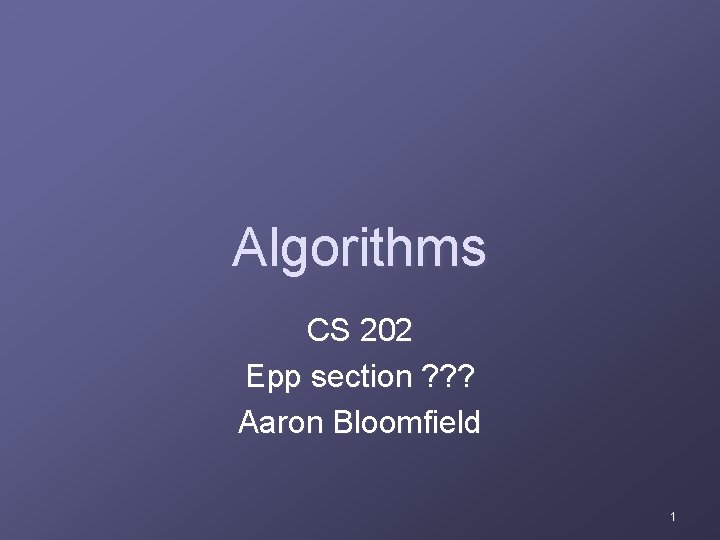Algorithms CS 202 Epp section ? ? ? Aaron Bloomfield 1What is an algorithm? An algorithm is “a finite set of precise instructions for performing a computation or for solving a problem” n A program is one type of algorithm All programs are algorithms Not all algorithms are programs! n n n Directions to somebody’s house is an algorithm A recipe for cooking a cake is an algorithm The steps to compute the cosine of 90° is an algorithm 2Some algorithms are harder than others Some algorithms are easy n n Finding the largest (or smallest) value in a list Finding a specific value in a list Some algorithms are a bit harder n Sorting a list Some algorithms are very hard n Finding the shortest path between Miami and Seattle Some algorithms are essentially impossible n Factoring large composite numbers In section 2. 2, we’ll see how to rate how “hard” algorithms are 3Algorithm 1: Maximum element Given a list, how do we find the maximum element in the list? To express the algorithm, we’ll use pseudocode n Pseudocode is kinda like a programming language, but not really 4Algorithm 1: Maximum element Algorithm for finding the maximum element in a list: procedure max (a 1, a 2, …, an: integers) max : = a 1 for i : = 2 to n if max < ai then max : = ai {max is the largest element} 5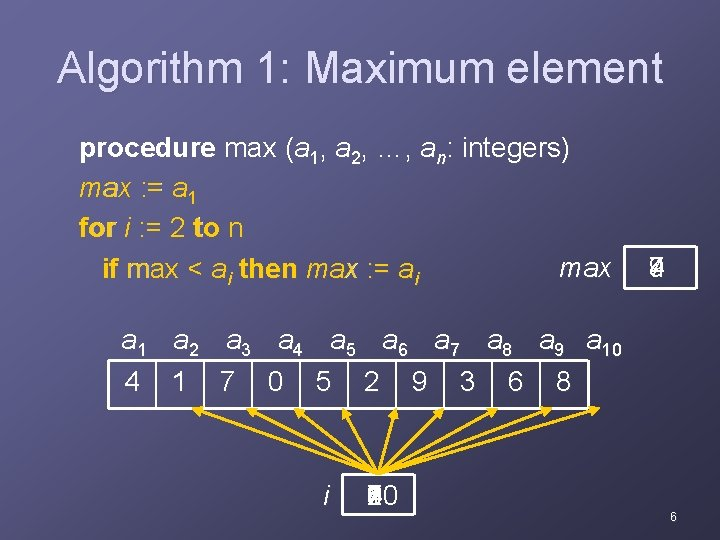Algorithm 1: Maximum element procedure max (a 1, a 2, …, an: integers) max : = a 1 for i : = 2 to n max if max < ai then max : = ai 9 7 4 a 1 a 2 a 3 a 4 a 5 a 6 a 7 a 8 a 9 a 10 4 1 7 0 5 2 9 3 6 8 i 10 9 8 7 6 5 4 3 2 6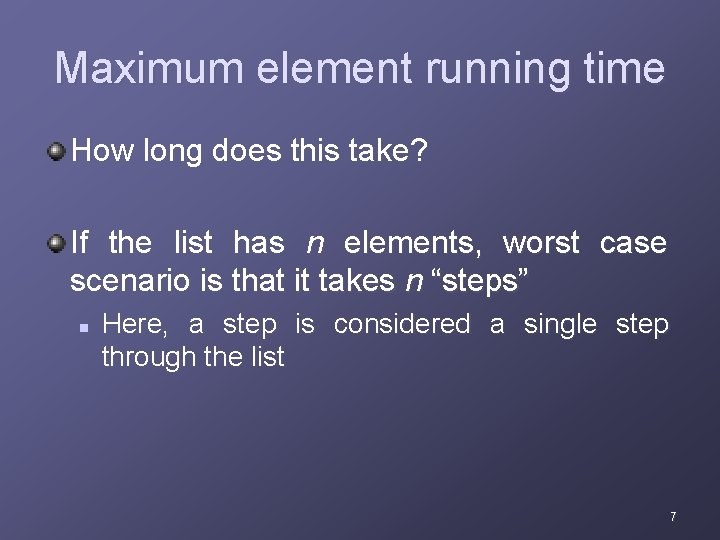Maximum element running time How long does this take? If the list has n elements, worst case scenario is that it takes n “steps” n Here, a step is considered a single step through the list 7Properties of algorithms Algorithms generally share a set of properties: n n n n Input: what the algorithm takes in as input Output: what the algorithm produces as output Definiteness: the steps are defined precisely Correctness: should produce the correct output Finiteness: the steps required should be finite Effectiveness: each step must be able to be performed in a finite amount of time Generality: the algorithm should be applicable to all problems of a similar form 8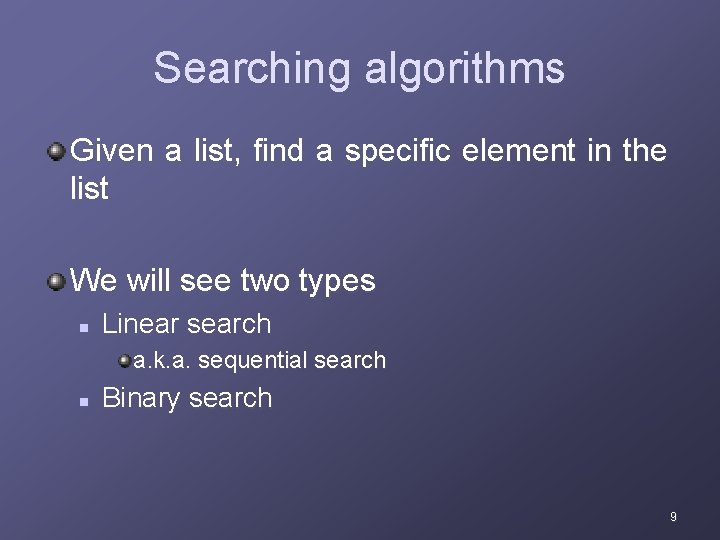Searching algorithms Given a list, find a specific element in the list We will see two types n Linear search a. k. a. sequential search n Binary search 9Algorithm 2: Linear search Given a list, find a specific element in the list n List does NOT have to be sorted! procedure linear_search (x: integer; a 1, a 2, …, an: integers) i : = 1 while ( i ≤ n and x ≠ ai ) i : = i + 1 if i ≤ n then location : = i else location : = 0 {location is the subscript of the term that equals x, or it is 0 if x is not found} 10Algorithm 2: Linear search, take 1 procedure linear_search (x: integer; a 1, a 2, …, an: integers) i : = 1 while ( i ≤ n and x ≠ ai ) x 3 i : = i + 1 if i ≤ n then location : = i location 8 else location : = 0 a 1 a 2 a 3 a 4 a 5 a 6 a 7 a 8 a 9 a 10 4 1 7 0 5 2 9 3 6 8 i 1 8 7 6 5 4 3 2 11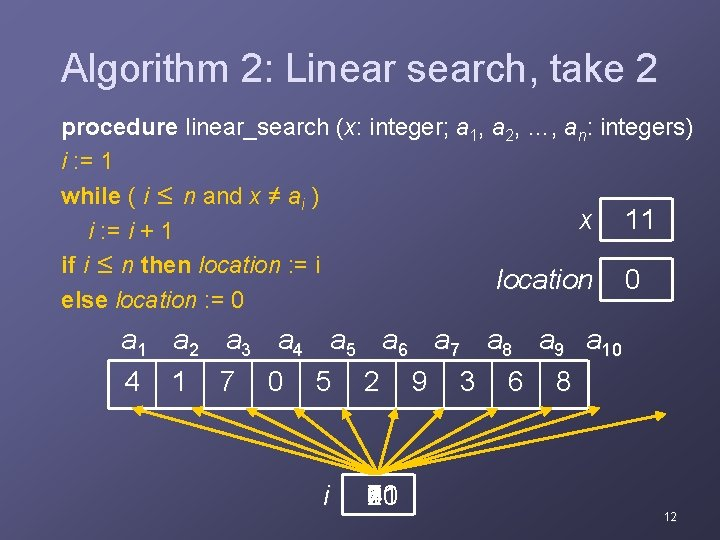Algorithm 2: Linear search, take 2 procedure linear_search (x: integer; a 1, a 2, …, an: integers) i : = 1 while ( i ≤ n and x ≠ ai ) x 11 i : = i + 1 if i ≤ n then location : = i location 0 else location : = 0 a 1 a 2 a 3 a 4 a 5 a 6 a 7 a 8 a 9 a 10 4 1 7 0 5 2 9 3 6 8 i 90 8 7 6 5 4 3 2 1 11 12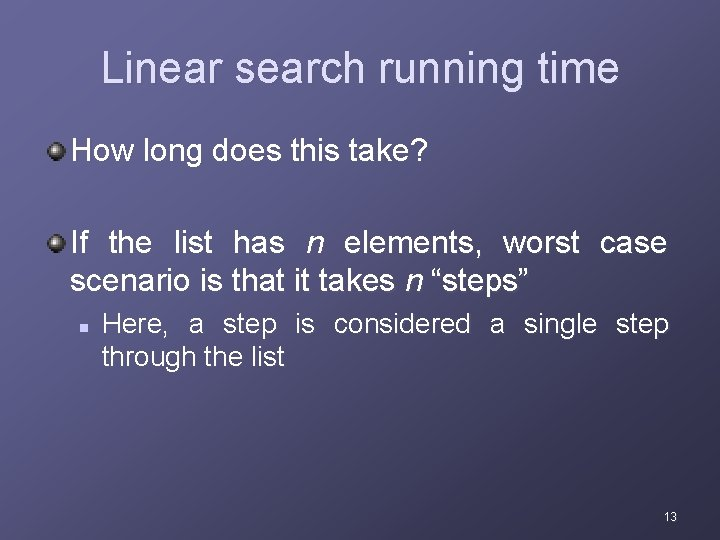Linear search running time How long does this take? If the list has n elements, worst case scenario is that it takes n “steps” n Here, a step is considered a single step through the list 13Algorithm 3: Binary search Given a list, find a specific element in the list n List MUST be sorted! Each time it iterates through, it cuts the list in half procedure binary_search (x: integer; a 1, a 2, …, an: increasing integers) i : = 1 { i is left endpoint of search interval } j : = n { j is right endpoint of search interval } while i < j begin m : = (i+j)/2 { m is the point in the middle } if x > am then i : = m+1 else j : = m end if x = ai then location : = i else location : = 0 {location is the subscript of the term that equals x, or it is 0 if x is not found} 14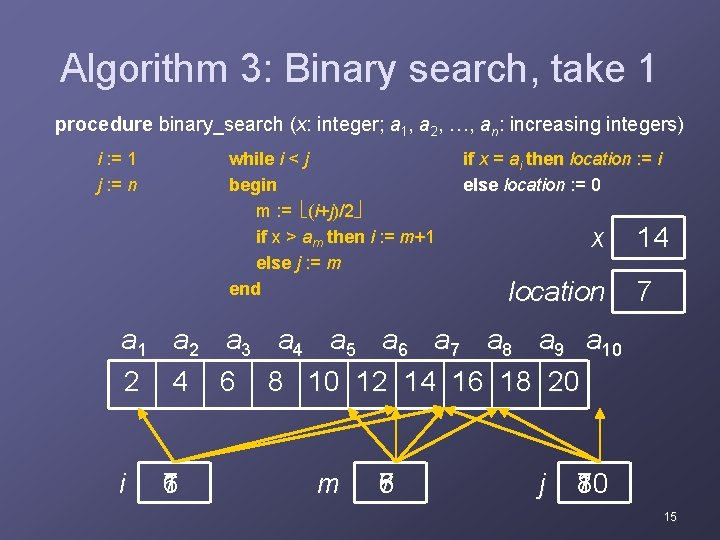Algorithm 3: Binary search, take 1 procedure binary_search (x: integer; a 1, a 2, …, an: increasing integers) i : = 1 j : = n while i < j begin m : = (i+j)/2 if x > am then i : = m+1 else j : = m end if x = ai then location : = i else location : = 0 x location 14 7 a 1 a 2 a 3 a 4 a 5 a 6 a 7 a 8 a 9 a 10 2 4 6 8 10 12 14 16 18 20 i 1 6 7 m 5 8 7 6 j 10 8 7 15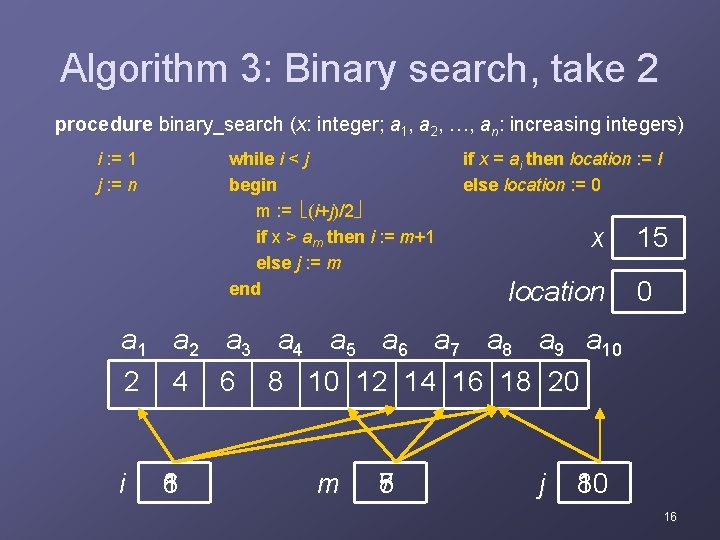Algorithm 3: Binary search, take 2 procedure binary_search (x: integer; a 1, a 2, …, an: increasing integers) i : = 1 j : = n while i < j begin m : = (i+j)/2 if x > am then i : = m+1 else j : = m end if x = ai then location : = Ii else location : = 0 x location 15 0 a 1 a 2 a 3 a 4 a 5 a 6 a 7 a 8 a 9 a 10 2 4 6 8 10 12 14 16 18 20 i 1 6 8 m 5 8 7 j 10 8 16Algorithm 3: Binary search A somewhat alternative view of what a binary search does… 17Binary search running time How long does this take (worst case)? If the list has 8 elements n It takes 3 steps If the list has 16 elements n It takes 4 steps If the list has 64 elements n It takes 6 steps If the list has n elements n It takes log 2 n steps 18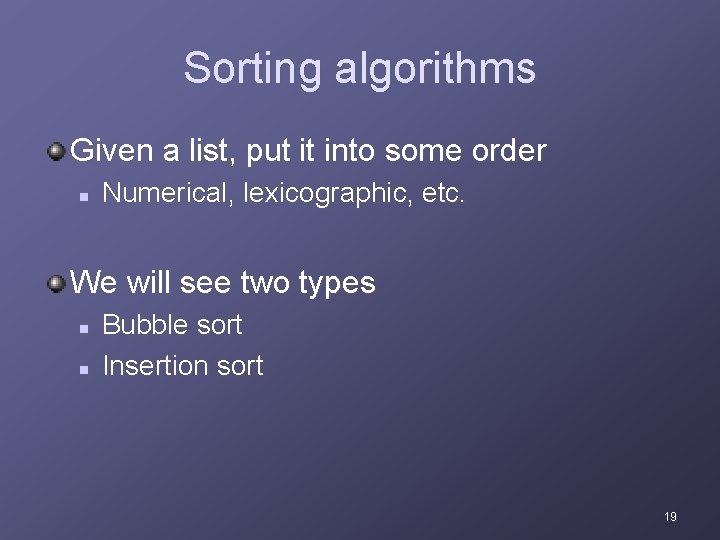Sorting algorithms Given a list, put it into some order n Numerical, lexicographic, etc. We will see two types n n Bubble sort Insertion sort 19Algorithm 4: Bubble sort One of the most simple sorting algorithms n Also one of the least efficient It takes successive elements and “bubbles” them up the list procedure bubble_sort (a 1, a 2, …, an) for i : = 1 to n-1 for j : = 1 to n-i if aj > aj+1 then interchange aj and aj+1 { a 1, …, an are in increasing order } 20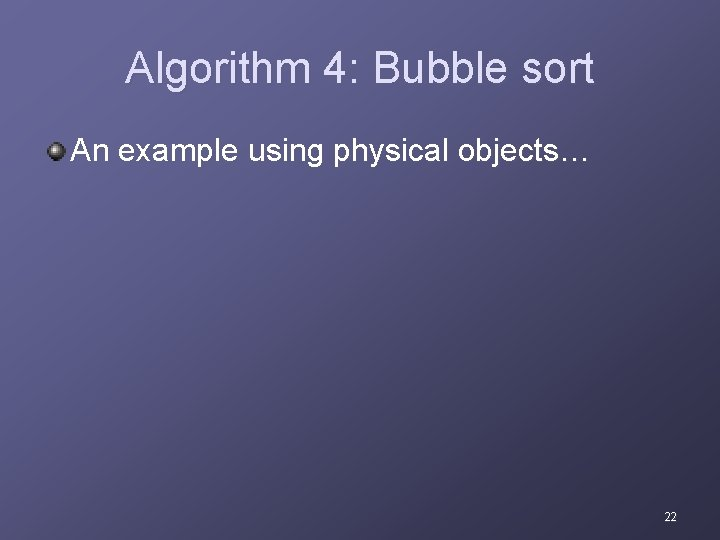Algorithm 4: Bubble sort An example using physical objects… 22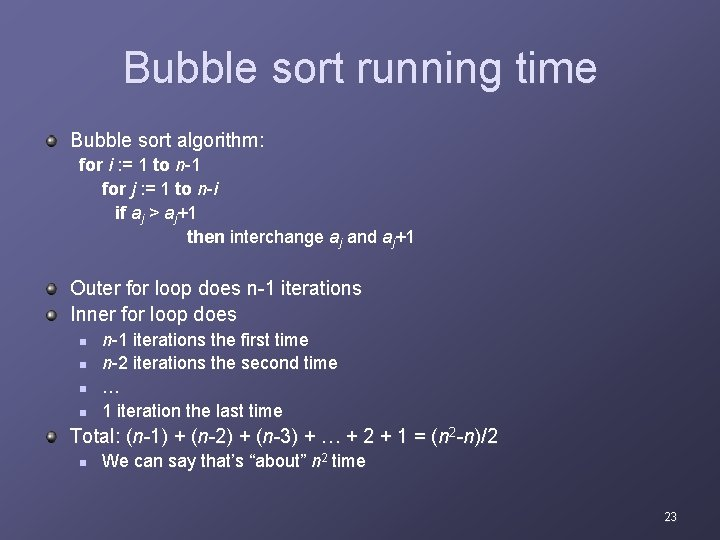Bubble sort running time Bubble sort algorithm: for i : = 1 to n-1 for j : = 1 to n-i if aj > aj+1 then interchange aj and aj+1 Outer for loop does n-1 iterations Inner for loop does n n n-1 iterations the first time n-2 iterations the second time … 1 iteration the last time Total: (n-1) + (n-2) + (n-3) + … + 2 + 1 = (n 2 -n)/2 n We can say that’s “about” n 2 time 23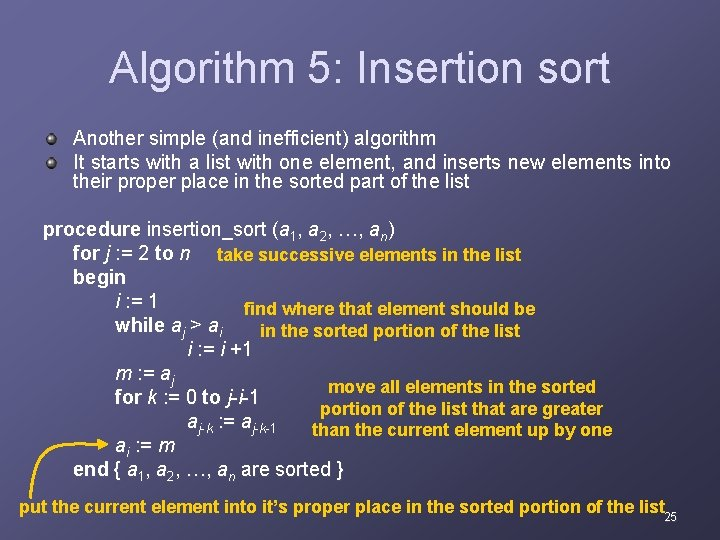Algorithm 5: Insertion sort Another simple (and inefficient) algorithm It starts with a list with one element, and inserts new elements into their proper place in the sorted part of the list procedure insertion_sort (a 1, a 2, …, an) for j : = 2 to n take successive elements in the list begin i : = 1 find where that element should be while aj > ai in the sorted portion of the list i : = i +1 m : = aj move all elements in the sorted for k : = 0 to j-i-1 portion of the list that are greater aj-k : = aj-k-1 than the current element up by one ai : = m end { a 1, a 2, …, an are sorted } put the current element into it’s proper place in the sorted portion of the list 25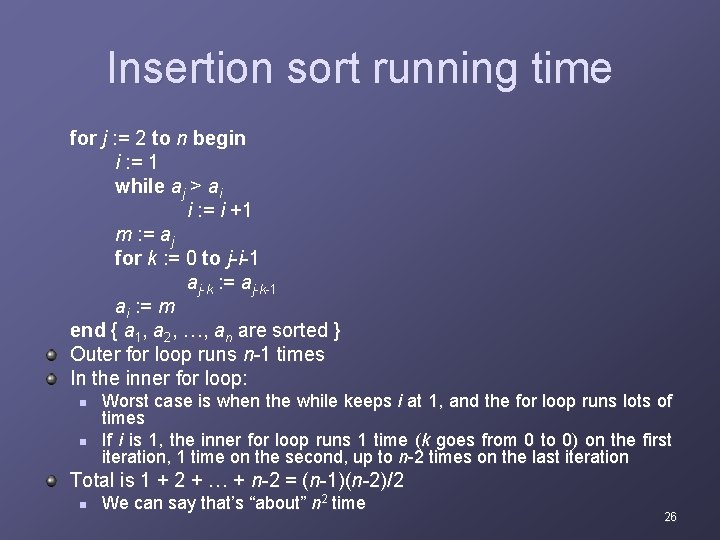Insertion sort running time for j : = 2 to n begin i : = 1 while aj > ai i : = i +1 m : = aj for k : = 0 to j-i-1 aj-k : = aj-k-1 ai : = m end { a 1, a 2, …, an are sorted } Outer for loop runs n-1 times In the inner for loop: n n Worst case is when the while keeps i at 1, and the for loop runs lots of times If i is 1, the inner for loop runs 1 time (k goes from 0 to 0) on the first iteration, 1 time on the second, up to n-2 times on the last iteration Total is 1 + 2 + … + n-2 = (n-1)(n-2)/2 n We can say that’s “about” n 2 time 26Comparison of running times Searches n n n Linear: n steps Binary: log 2 n steps Binary search is about as fast as you can get Sorts n n n Bubble: n 2 steps Insertion: n 2 steps There are other, more efficient, sorting techniques In principle, the fastest are heap sort, quick sort, and merge sort These each take n * log 2 n steps In practice, quick sort is the fastest, followed by merge sort 27